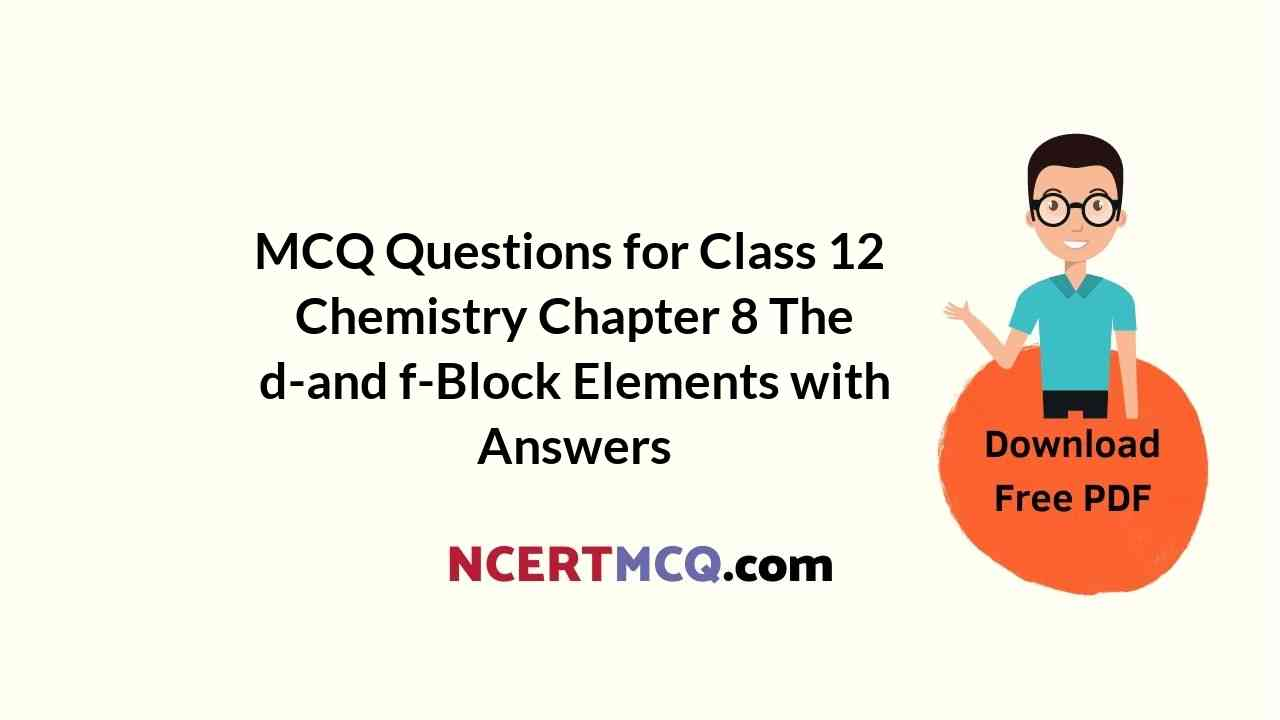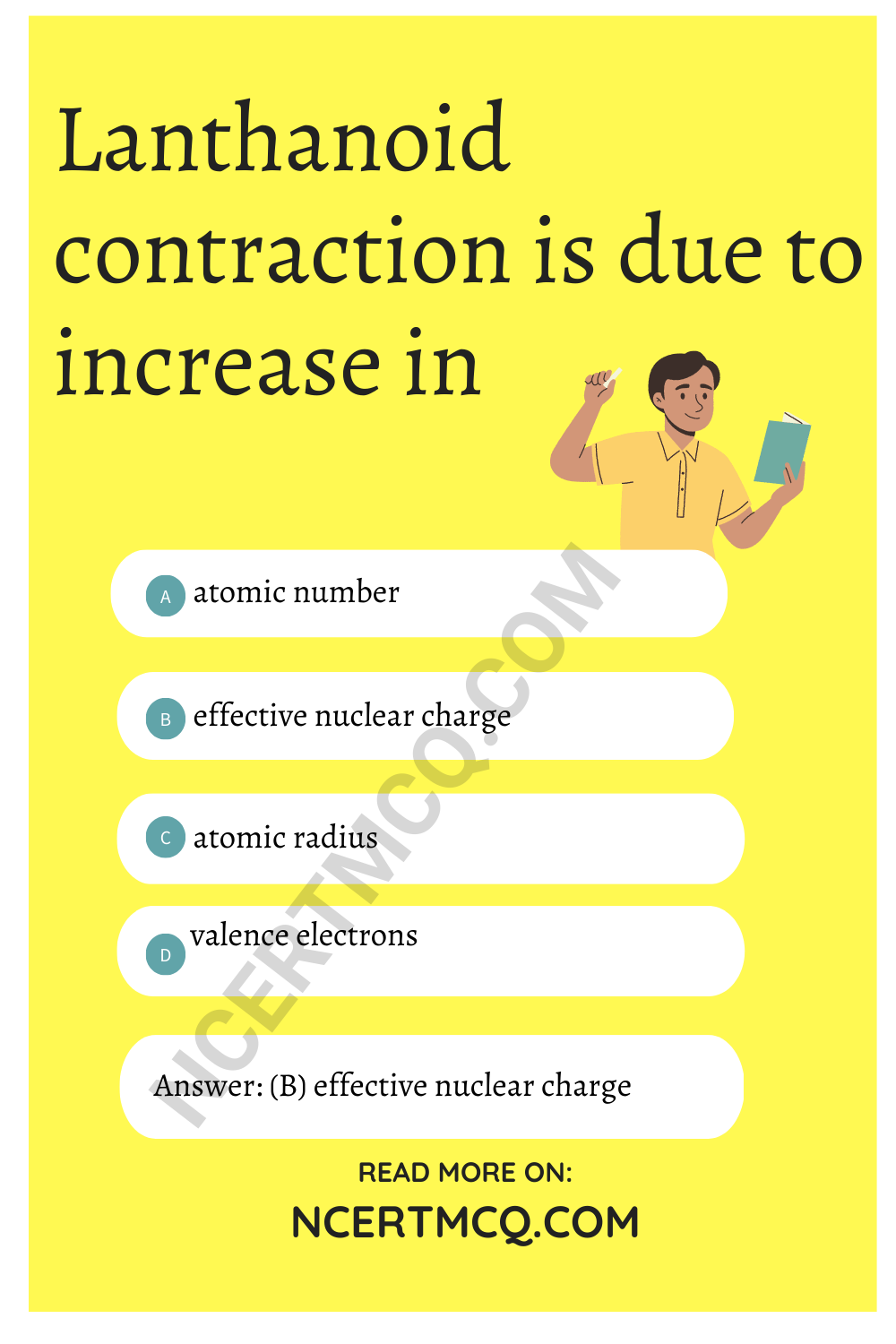Check the below NCERT MCQ Questions for Class 12 Chemistry Chapter 8 The d-and f-Block Elements with Answers Pdf free download. MCQ Questions for Class 12 Chemistry with Answers were prepared based on the latest exam pattern. We have provided The d-and f-Block Elements Class 12 Chemistry MCQs Questions with Answers to help students understand the concept very well.

## The d-and f-Block Elements Class 12 MCQs Questions with Answers

D And F Block Elements Class 12 MCQ Question 1.
Oxidation number of gold metal is
(a) +1
(b) 0
(c) -1
(d) all of these

MCQ On D And F Block Elements Question 2.
Shape of d-orbital is
(a) spherical
(b) dumb bell
(c) double dumb bell
(d) none of these

D And F Block Elements MCQ Question 3.
Electronic configuration of alkaline earth element is
(a) ns2
(b) ns1
(c) np6
(d) ns0

D And F Block Elements MCQ Pdf Question 4.
Lanthanoid contraction is due to increase in
(a) atomic number
(b) effective nuclear charge
(d) valence electronsWhich one of the following is called green vitriol?
(a) FeSO4 7H2O
(b) CuSO4 5H2O
(c) CaSO4 2H2O
(d) None of these

D Block Elements MCQ Questions Question 6.
Which block of elements are known as transition elements?
(a) p-block
(b) s-block
(c) d-block
(d) f-block

D-Block Elements MCQs With Answers Question 7.
Most abundant element in earth’s crust is
(a) Si
(b) Al
(c) Zn
(d) Fe

MCQ Of D And F Block Elements Question 8.
Which one of the following is diamagnetic ion?
(a) Co2+
(b) Ni2+
(c) Cu2+
(d) Zn2+

MCQ On F Block Elements Question 9.
Which of the following is not an element of first transition series?
(a) Iron
(b) Chromium
(c) Magnesium
(d) Nickel

MCQ On D Block Elements Question 10.
The correct order of E$$_{0}^{M^{2+}/M}$$ values with negative sign for the four successive elements Cr, Mn, Fe and Co is
(a) Fe > Mn > Cr > Co
(b) Cr > Mn > Fe > Co
(c) Mn > Cr > Fe > Co
(d) Cr > Fe > Mn > Co

Answer: (c) Mn > Cr > Fe > Co

MCQs On D And F Block Elements Question 11.
The number of unpaired electrons in gaseous species of Mn3+, Cl3+ and V3+ respectively are and the most stable species is
(a) 4, 3 and 2; V3+
(b) 3, 3 and 2; Cr3+
(c) 4, 3 and 2; Cr3+
(d) 3, 3 and 3; Mn3+

Answer: (c) 4, 3 and 2; Cr3+

D Block MCQ Class 12 Question 12.
Fe3+ ion is more stable than Fe2+ ion because
(a) more the charge on the atom, more is its stability
(b) configuration of Fe2+ is 3d5 while Fe3+ is 3d5
(c) Fe2+ has a larger size than Fe3+
(d) Fe3+ ions are coloured hence more stable

Answer: (b) configuration of Fe2+ is 3d5 while Fe3+ is 3d5

D Block Elements MCQ Question 13.
Colour of transition metal ions are due to absorption of some wavelength. This results in
(a) d-s transition
(b) s-s transition
(c) s-t/transition
(d) d-d transition

MCQs On D Block Elements Question 14.
Which group contains coloured ions out of the following?
1. Cu. 2. Ti4- 3. Co2+ 4. Fe2-
(a) 1, 2, 3, 4
(b) 3, 4
(c) 2, 3
(4) 1, 2

D And F Block Elements MCQs With Answers Question 15.
The melting points of Cu, Ag and Au follow the order
(a) Cu > Ag > Au
(b) Cu >Au > Ag
(c) Au > Ag > Cu
(d) Ag > Au > Cu

Answer: (b) Cu >Au > Ag

Question 16.
In which of the following pairs of ions, the lower oxidation state in aqueous solution is more stable than the other?
(a) Ti+, Ti3+
(b)Cu+, Cu3+
(c) Cr2+, Cr3+
(d) V2+, VO2+ (V4)

Question 17.
Which of the following pairs of ions have the same electronic configuration?
(a) Cu2+, Cr2+
(b) Fe3+, Mn2+
(c) Co3+, Ni3+
(d) Sc3+, Cr3+

Question 18.
The melting point of copper is higher than that of zinc because
(a) copper has a bcc structure
(b) the atomic volume of copper is higher
(c) the electrons of copper are involved in metallic bonding
(d) the s as well as d electrons of copper are involved in metallic bonding

Answer: (c) the electrons of copper are involved in metallic bonding

Question 19.
Zr and Hf have almost equal atomic and ionic radii because of
(a) diagonal relationship
(b) lartthanoid contraction
(c) actinoid contraction
(d) belonging to the same group

Question 20.
Which of the following is not an amphoteric ion?
(a) Al3+
(b) Cr3+
(c) Fe3+
(d) Zn2+

Question 21.
What happens when potassium iodide reacts with acidic solution of potassium dichromate?
(a) It liberates iodine
(b) Potassium sulphate is formed
(c) Chromium sulphate is formed
(d) All the above products are formed

Answer: (d) All the above products are formedQuestion 22.
The number of moles of KMnO4 that are needed to react completely with one mole of ferrous oxalate in acidic solution is
(a) 3/5
(b) 2/5
(c) 4/5
(d) 1

Question 23.
Acidified potassium dichromate reacts with potassium iodide and oxidises it to I2. What is the oxidation state of chromium in the products of the reaction?
(a) +4
(b) +6
(c) +3
(d) +2

Question 24.
One mole of acidfied K2Cr3+O on reaction with excess KI will liberate ………….. mole(s) of I2.
(a) 6
(b) 1
(c) 7
(d) 2

Question 25.
In the diehromateanion (Cr2O$$_{7}^{2-}$$)
(a) all Cr – O bonds are equivalent
(b) 6 Cr – O bonds are equivalent
(c) 3 Cr – O bonds are equivalent
(d) no bonds in Cr2O$$_{7}^{2-}$$ are equivalent